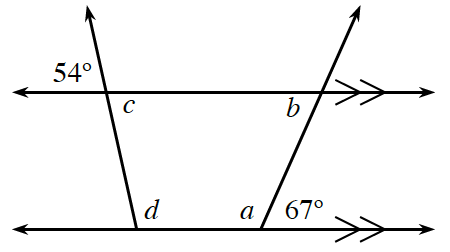### Home > INT3 > Chapter Ch4 > Lesson 4.1.2 > Problem4-26

4-26.Given the figure at right, determine the measures of the four lettered angles. Explain how you found each angle measure and name the relationship(s) you used to do so. Homework Help ✎

Use your knowledge about supplementary angles, vertical angles, alternate interior angles, same side interior angles, and parallel lines.

$a=113°$
$c=54°$
$b=67°$
$d=126°$